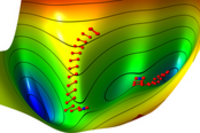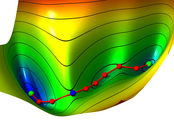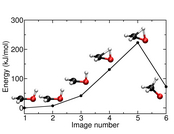DL-FIND

A Geometry Optimizer for Atomistic Simulation Codes

A geometry optimization library for quantum chemical and QM/MM calculations to be included into electronic structure codes.

DL-FIND can be used to search for minima, transition states (the main strength of the code), and conical intersections.Trajectories of two transition-state searches using the dimer method in DL-FIND. The dimer midpoint converges to the transition state (blue sphere).

Reference

All work totally or partially based on DL-FIND should cite  as well as the original references of the optimization algorithms used (references given in ).

Description

A light-weighted description of geometry optimization in general and DL-FIND in particular can be found in Frontiers 2007.

Functionality

Coordinate systems

• Cartesian coordinates (including frozen atoms and components), mass-weighted Cartesian coordinates
• Internal coordinates (including all constraints):
• DLC (delocalized internal coordinates, i.e. redundant internal coordinates)
• DLC-TC (total connection)
• HDLC (hybrid delocalized internal coordinates, see Phys. Chem. Chem. Phys. 2, 2177 (2000))
• HDLC-TC

Combinations of coordinates (images):

• Dimer method 
• NEB (nudged elastic band) 

All of the combinations work with all versions of coordinate systems.

Optimizers

• steepest descent
• L-BFGS
• P-RFO, Hessian update mechanisms: Powell and Bofill. Hessian either by input or by finite-difference. In the latter case either in Cartesians (then the update also in Cartesians, and one can output frequencies), or in internals.
• Damped dynamics
• Algorithms for Conical intersection search:
• Penalty function
• Gradient projection method
• Lagrange-Newton method
• Stochastic search methods (including a genetic algorithm) for global and local minimization. These methods optimize by calculating may energies in parallel and are thus well-suited for massively parallel computation.Converged nudged-elastic band path on an example surface (Müller-Brown potential). The green spheres indicate minima, the blue sphere indicates the climbing image which converged to the transition state.

Line search algorithms

• Simple scaling of the proposed step (covering the maximum step length)
• Trust radius based on energy decrease
• Trust radius based on the projection of the gradient on the step

The design allows new methods to be easily implemented.

Reaction rate calculations with or without tunneling contributions

• Instanton theory (aka imaginary-F theory or harmonic quantum transition state theory) to calculate tunneling rates.
• Instanton optimizations with a quadratically-converging optimizer 
• Instanton rate calculations (parallelized)
• Adaptive step size in instanton calculations 
• Reaction rates without tunnelingEnergy and geometries of a nudged-elastic band path of a simple chemical system, optimized with DL-FIND.

Other functionality

• The optimizer is fully restartable.
• DL-FIND can be included in ChemShell and GAMESS-UK and was used in conjuction with a nuber of other codes

Authors

• Johannes Kästner, main author
• Tom W. Keal contributed conical intersection search algorithms, parallelization of NEB and finite-difference Hessian calculations and fixed many bugs.
• Joanne M. Carr is adding parallel search algorithms
• Judith B. Rommel contributed to the implementation of instanton theory
• Salomon Billeter and Alexander Turner: parts of their HDLCopt routines have been used in the coordinate transformation, by courtesy of the Max-Planck-Institute for coal research.
• The L-BFGS code by Jorge Nocedal was used